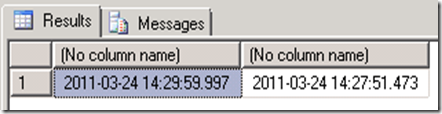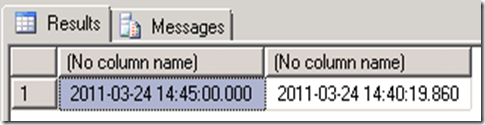## Thursday, March 24, 2011

### SQL Server: Rounding DateTime to Intervals

There are times when you are wanting to produce bar charts that you wish to round times to the quarter hour and keep it as a time. The typical solution is to cast as a varchar() and use the string, unfortunately this works well only for some intervals. Doing this properly is not hard, but you need to be careful not to have numeric calculation artifacts creeping in. Below, I am trying to round everything to the closest quarter hour, other periods just require some change of numbers.

• 15 minutes –> 24 x 4 =96 Intervals
• 10 minutes –> 24 x 6 = 144 Intervals
• 1 minute –> 24 x 60 =1440 Intervals.

### The Oops not perfect solution

The function below seems to create the needed result – until you test it and discover that the times are not always precise (arithmetic approximation  issues). This can cause problems with some usages.

```ALTER FUNCTION QtrHr
(
@When DateTime
)
RETURNS DateTime
AS
BEGIN
DECLARE @est numeric(38,30)
Set @est=Round(Cast(@When as numeric(38,30)) * 96.0,0)/96.0
SET @When= Cast(@est as DateTime)
RETURN @When
END
GO
select dbo.QtrHr(GetDate()),GetDate()

```### A more reliable approach

I rewrote the function to eliminate reliance on mathematical functions being precise. I grab the day part and then the number of 15 minute intervals, combining them with the DateAdd function. Everything now works fine.

```ALTER FUNCTION QtrHr
(
@When DateTime
)
RETURNS DateTime
AS
BEGIN
DECLARE @est numeric(38,30)
Declare @day int
Declare @min int
Set @est=Round(Cast(@When as numeric(38,30)) * 96.0,0)
SET @Day=@est/96.0
Set @Min=(@est % 96)*15